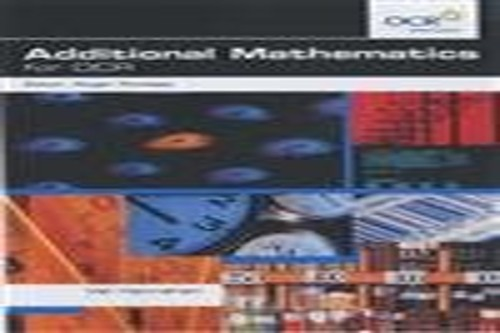Additional Mathematics for OCR by Val Hanrahan

Teach with confidence, knowing your students will be fully prepared for their exams, with this detailed textbook, endorsed by OCR.

6 in stock

Additional Mathematics for OCR Summary

Additional Mathematics for OCR by Val Hanrahan

Teach with confidence, knowing your students will be fully prepared for their exams, with this detailed textbook that is closely tailored to the specification and has been endorsed by OCR.

Endorsed by OCR for use with the OCR Additional Mathematics specification. This level 3 qualification in Key Stage 4 enables students to study higher level mathematics without having to embark on their AS modules.

- Accessible and concise, written by experienced authors to guide and encourage your higher level students towards success

- Includes an introduction to each topic followed by worked examples with commentaries

- Provides plenty of practice with hundreds of questions

- Ideal for students considering maths at AS/A level, accelerating their progress and aiding their future choices

1. Algebra I - review
2. Albebra II - techniques
3. Algebra III - polynomials
4. Algebra IV - applications
5. Co-ordinate geometry I
6. Co-ordinate geometry II - applications7. Trigonometry I
8. Trigonometry II - applications
9. Calculus I - differentiation
10. Calculus II - integration
11. Calculus III - applications to kinematics

Why buy from World of BooksOur excellent value books literally don't cost the earthFree delivery in the USEvery used book bought is one saved from landfill

Additional Mathematics for OCR Reviews

...it contains all the information you'll need for the course as well as a HUGE number of questions for practice and makes it all very interesting. * Amazon reviewer *
This is the officially endorsed course text and as such represents the best text book offering for the Additional Maths OCR qualification. It covers all of the topics in a clear fashion and is well presented. However, I would say that it needs more questions for practice and it lacks some of the more challenging, quirky-style of problem which candidates will meet on the examination. Overall it is a good text book but will need to be supplemented with other material (such as the practice book!). -- Mr Stephen Black * Abigail Lambert *

Val Hanrahan has been Head of Maths at an Independent school. She has also written several Pure Maths A/AS texts.
Roger Porkess has written and edited books for GCSE, A/AS level and Key Stage 3.

• : 1 Algebra: review
• : Linear expressions
• : Solving linear equations
• : Changing the subject of an equation
• : Quadratic expressions
• : Solving a quadratic equation that factorises
• : Completing the square
• : Simultaneous equations
• : 2 Algebra: techniques
• : Linear inequalities
• : Solving quadratic inequalities
• : Manipulating algebraic fractions
• : Solving equations involving fractions
• : Simplifying expressions containing square roots
• : 3 Algebra: polynomials
• : Operations with polynomials
• : The Factor Theorem
• : The Remainder Theorem
• : 4 Algebra: applications
• : The binomial expansion
• : The binomial distribution
• : 5 Co-ordinate geometry I
• : Co-ordinates
• : The gradient of a line
• : Parallel and perpendicular lines
• : The distance between two points
• : Midpoint of a line joining two points
• : The equation of a straight line
• : Drawing a line given its equation
• : Finding the equation of a line
• : Intersection of two lines
• : The circle
• : 6 Co-ordinate geometry II, applications
• : Inequalities
• : Using inequalities for problem solving
• : 7 Trigonometry I
• : Using trigonometry in right angled triangles
• : Trigonometrical functions for angles of any size
• : Solving trigonometrical equations
• : Identities involving sin , cos , and tan
• : The area of a triangle
• : The Sine and Cosine rules
• : 8 Trigonometry II - Applications
• : Applications of the Sine and Cosine rules in 2-D
• : Height and distance problems in 3-D
• : 3-D problems involving solids
• : 9 Calculus I - Differentiation
• : Finding the gradient of a curve
• : Differentiating by using standard results
• : Tangents and normals
• : Stationary points
• : Curve sketching
• : 10 Calculus II - Integration
• : Reversing differentiation
• : Using integration to find areas
• : 11 Calculus III - Applications to kinematics
• : Variable acceleration problems
• : The formulae for constant acceleration

GOR001221067
Additional Mathematics for OCR by Val Hanrahan
Val Hanrahan
Used - Very Good
Paperback
Hodder Education
2004-02-27
272
0340869607
9780340869604
N/A
Book picture is for illustrative purposes only, actual binding, cover or edition may vary.
This is a used book - there is no escaping the fact it has been read by someone else and it will show signs of wear and previous use. Overall we expect it to be in very good condition, but if you are not entirely satisfied please get in touch with us.